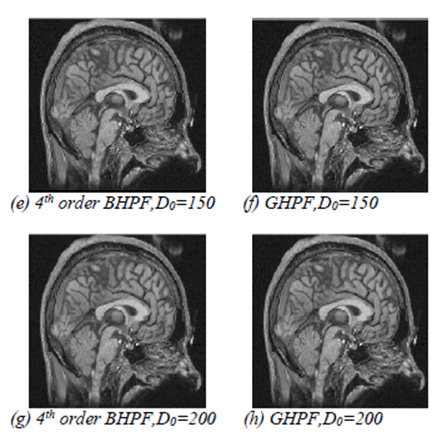Figure 3: Image sharpening by GHPF and 4th order BHPF with D0=50, 100, 150, 200. (a) 4th order BHPF, D0=50, (b) GHPF, D0=50, (c) 4th order BHPF, D0=100, (d) GHPF, D0=100, (e) 4th order BHPF, D0=150, (f) GHPF, D0=150, (g) 4th order BHPF, D0=200, (h) GHPF, D0=200.